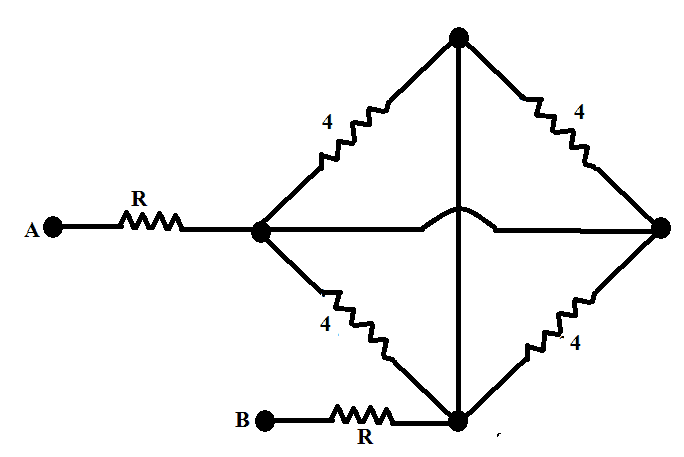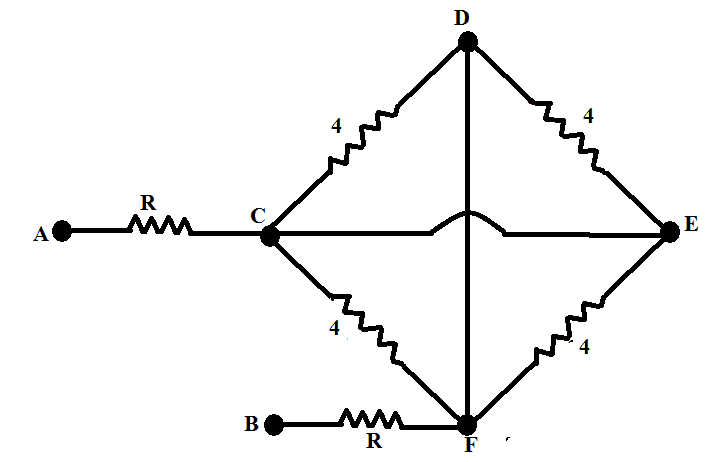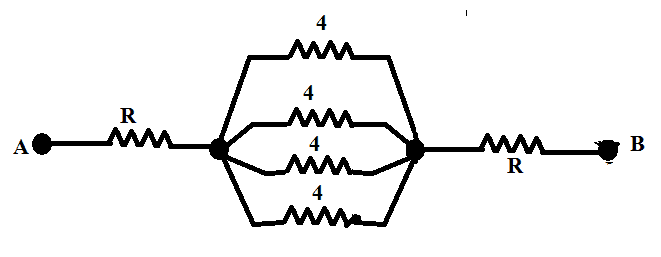# Equivalent resistance

• arpon

## Homework StatementCalculate the equivalent resistance between A and B.[/B]

## The Attempt at a Solution

I solved this problem by using wye-delta transformation. Is there any other method to solve this? The speciality in this circuit, I noticed, two of resistances are 0.[/B]

Yes there is. Look at the square on the right. They try to confuse you by drawing it this way, but if you flip down the upper half, you see that the four 4 ##\Omega## resistors are in fact all ...

•arpon
Yes there is. Look at the square on the right. They try to confuse you by drawing it this way, but if you flip down the upper half, you see that the four 4 ##\Omega## resistors are in fact all ...So, I can think C and E as the same point. D and F are the same point too. So, I can change this circuit intothis one:Am I right?

That's the idea, yes.

•arpon